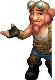X Axis min and max value issueTop achievementsRank 1
Richard asked on 25 May 2011, 04:08 PM
Hi

Iam having trouble being able to assign a min and max value to my x axis. I have read multiple posts stating that you must set the following to enable you to define a min and max value which i define is server side code (vb.net):

XAxis.AutoScale = False
XAxis.MinValue = 1
XAxis.MaxValue = 120
XAxis.Step = 10

I have also tried using this instead of the above:

XAxis.AutoScale = False

This is my mark up:

`<``telerik:RadChart` `ID``=``"RadChart1"` `runat``=``"server"`
`        ``Height``=``"100px"`
`        ``Width``=``"180px"`
`        ``ChartTitle-Visible``=``"false"`
`        ``Legend-Visible``=``"false"`
`        ``SeriesOrientation``=``"Horizontal"` `>`
`  ``</``telerik:RadChart``>`

I define a datasource using a list of my custom objects which has 2 decimal values (depth - for the x axis, value - for y axis) and then i databind it.

My current list has 3 items,
Item 1: depth 1, value 50
item 2: depth 2, value 10
item 3: depth 3, value 30

Attached is the results:

I would like the xaxis (note horizontal series orientation - so the left axis) to display min value 1 and max value 120. How can i achieve then, what am i missing?

Cheers

Richard.

## 4 Answers, 1 is accepted

0Gimmik
Top achievementsRank 1
answered on 25 May 2011, 08:10 PM
Hi Richard,

The following code worked for me.

`RadChart1.PlotArea.XAxis.AutoScale = ``false``;`
`RadChart1.PlotArea.XAxis.MinValue = 1;`
`RadChart1.PlotArea.XAxis.MaxValue = 120;`
`RadChart1.PlotArea.XAxis.Step = 10;`

Can you post a runnable example so I can see what is going wrong?

Thanks,
-Gimmik
0Richard
Top achievementsRank 1
answered on 26 May 2011, 01:45 AM
`Imports System.Drawing`
`Imports Telerik.Charting`

`Partial Public Class _Default`
`    ``Inherits System.Web.UI.Page`

`    ``Protected Sub Page_Load(ByVal sender As Object, ByVal e As System.EventArgs) Handles Me.Load`

`        ``Dim lstGammaDetails As New List(Of GammaDetail)`

`        ``lstGammaDetails = GetGammaDetails()`

`        ``RadChart1.Series.Clear()`

`        ``'Chart appearance`
`        ``RadChart1.Height = Unit.Pixel(300)`
`        ``RadChart1.PlotArea.Appearance.FillStyle.FillType = Telerik.Charting.Styles.FillType.Solid`
`        ``RadChart1.PlotArea.Appearance.FillStyle.MainColor = Color.White`
`        ``RadChart1.PlotArea.Appearance.Dimensions.Margins.Top = New Telerik.Charting.Styles.Unit(28)`
`        ``RadChart1.PlotArea.Appearance.Dimensions.Margins.Left = New Telerik.Charting.Styles.Unit(40)`
`        ``RadChart1.PlotArea.Appearance.Dimensions.Margins.Right = New Telerik.Charting.Styles.Unit(8)`
`        ``RadChart1.PlotArea.Appearance.Dimensions.Margins.Bottom = New Telerik.Charting.Styles.Unit(10)`
`        ``RadChart1.IntelligentLabelsEnabled = False`
`        ``RadChart1.PlotArea.XAxis.Visible = Styles.ChartAxisVisibility.True`

`        ``'X Axis`
`        ``RadChart1.PlotArea.XAxis.DataLabelsColumn = "Depth"`
`        ``RadChart1.PlotArea.XAxis.AutoScale = False`
`        ``RadChart1.PlotArea.XAxis.LayoutMode = Styles.ChartAxisLayoutMode.Normal`

`        ``'Min and Max values `
`        ``RadChart1.PlotArea.XAxis.MinValue = 1`
`        ``RadChart1.PlotArea.XAxis.MaxValue = 120`
`        ``RadChart1.PlotArea.XAxis.Step = 1`

`        ``'RadChart1.PlotArea.XAxis.AddRange(1, 120, 1)`

`        ``'Y Axis2`
`        ``RadChart1.PlotArea.YAxis2.Appearance.MajorGridLines.Visible = True`
`        ``RadChart1.PlotArea.YAxis2.Appearance.MajorGridLines.Color = Color.Gray`
`        ``RadChart1.PlotArea.YAxis2.AxisLabel.TextBlock.Appearance.TextProperties.Color = Color.Black`
`        ``RadChart1.PlotArea.YAxis2.Appearance.TextAppearance.TextProperties.Color = Color.Black`

`        ``'Line series`
`        ``Dim chartSeries As New ChartSeries`
`        ``chartSeries.Appearance.LabelAppearance.Visible = False`
`        ``chartSeries.Name = "GAMMA"`
`        ``chartSeries.Type = ChartSeriesType.Line`
`        ``chartSeries.Appearance.LineSeriesAppearance.Color = System.Drawing.Color.LightBlue`
`        ``chartSeries.DataYColumn = "Value"`
`        ``chartSeries.YAxisType = ChartYAxisType.Secondary`

`        ``RadChart1.Series.Add(chartSeries)`
`        ``RadChart1.DataSource = lstGammaDetails`
`        ``RadChart1.DataBind()`

`    ``End Sub`

`    ``Private Function GetGammaDetails()`

`        ``Dim lstGD As New List(Of GammaDetail)`

`        ``Dim gd1 = New GammaDetail()`
`        ``gd1.Depth = 3`
`        ``gd1.Value = 30`
`        ``lstGD.Add(gd1)`

`        ``Dim gd2 = New GammaDetail()`
`        ``gd2.Depth = 2`
`        ``gd2.Value = 10`
`        ``lstGD.Add(gd2)`

`        ``Dim gd3 = New GammaDetail()`
`        ``gd3.Depth = 1`
`        ``gd3.Value = 50`
`        ``lstGD.Add(gd3)`

`        ``Return lstGD`

`    ``End Function`

`End Class`

`Public Class GammaDetail`

`    ``Private _depth As Decimal`
`    ``Public Property Depth() As Decimal`
`        ``Get`
`            ``Return _depth`
`        ``End Get`
`        ``Set(ByVal value As Decimal)`
`            ``_depth = value`
`        ``End Set`
`    ``End Property`

`    ``Private _value As Decimal`
`    ``Public Property Value() As Decimal`
`        ``Get`
`            ``Return _value`
`        ``End Get`
`        ``Set(ByVal value As Decimal)`
`            ``_value = value`
`        ``End Set`
`    ``End Property`

```End Class ```

`<%@ Page Language="vb" AutoEventWireup="false" CodeBehind="Default.aspx.vb" Inherits="RadChartMinMaxValue._Default" %>`

`<%@ Register Assembly="Telerik.Web.UI" Namespace="Telerik.Web.UI" TagPrefix="telerik" %>`
`<!DOCTYPE html PUBLIC "-//W3C//DTD XHTML 1.0 Transitional//EN" "http://www.w3.org/TR/xhtml1/DTD/xhtml1-transitional.dtd">`

`<``html` `xmlns``=``"http://www.w3.org/1999/xhtml"` `>`
`<``head` `runat``=``"server"``>`
`    ``<``title``></``title``>`
`</``head``>`
`<``body``>`
`    ``<``form` `id``=``"form1"` `runat``=``"server"``>`

`    ``</``form``>`
`        ``<``telerik:RadChart` `ID``=``"RadChart1"` `runat``=``"server"`
`                ``Height``=``"100px"`
`                ``Width``=``"250px"`
`                ``ChartTitle-Visible``=``"false"`
`                ``Legend-Visible``=``"false"`
`                ``SeriesOrientation``=``"Horizontal"` `BorderWidth``=``"0px"` `>`
`        ``</``telerik:RadChart``>`
`</``body``>`
`</``html``>`

0
AcceptedGimmik
Top achievementsRank 1
answered on 26 May 2011, 05:59 PM
Hi Richard,

I'm still researching exactly what is causing this issue - but for the time being here is a work-around.

If you comment out the line

`RadChart1.PlotArea.XAxis.DataLabelsColumn = ``"Depth"`

The chart seems to display correctly. I can't find much information the "`DataLabelsColumn" `property, but it seems to override other properties in the X-Axis.

Hope this helps,
-Gimmik
0Richard
Top achievementsRank 1
answered on 27 May 2011, 01:40 AM
Hi Gimmik

Thank you for the work around, I can now see my min and max values.

RichardRichard
Top achievementsRank 1Rank 1Rank 1